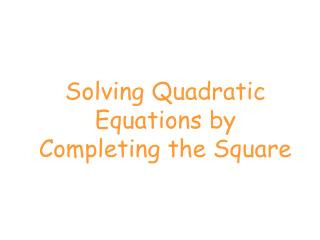DownloadDownload PresentationSolving Quadratic Equations by Completing the Square

# Solving Quadratic Equations by Completing the Square

Télécharger la présentation## Solving Quadratic Equations by Completing the Square

- - - - - - - - - - - - - - - - - - - - - - - - - - - E N D - - - - - - - - - - - - - - - - - - - - - - - - - - -
##### Presentation Transcript

1. Solving Quadratic Equations by Completing the Square

2. Solving Quadratic Equations by Completing the Square Solve the following equation by completing the square: Step 1: Isolate the constant

3. Solving Quadratic Equations by Completing the Square Step 2: Find the term that completes the square on the left side of the equation. It is half the coefficient of the linear term squared. Add that term to both sides.

4. Solving Quadratic Equations by Completing the Square Step 3: Factor the perfect square trinomial on the left side of the equation. Simplify the right side of the equation.

5. Solving Quadratic Equations by Completing the Square Step 4: Take the square root of each side

6. Solving Quadratic Equations by Completing the Square Step 5: Set up the two possibilities and solve

7. Let's solve another one by completing the square. 25 25 Since it doesn't factor get the constant on the other side ready to complete the square. So what do we add to both sides? the middle term's coefficient divided by 2 and squared Factor the left hand side Square root both sides (remember ) Subtract 5 from both sides to get x alone

8. Let's solve another one by completing the square. To complete the square we want the coefficient of the x2 term to be 1. 2 2 2 2 Divide everything by 2 16 16 Since it doesn't factor get the constant on the other side ready to complete the square. So what do we add to both sides? the middle term's coefficient divided by 2 and squared Factor the left hand side Square root both sides (remember ) Add 4 to both sides to get x alone

9. You try: • x2 - 8x + 3 = 0 • x2 -10x +7 = 0 • 5x2 – 20x -71 = -6 • 2x2 – 8x – 29 = -9